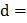# The maximum number of possible interference maxima for slit-separation equal to twice the wavelength is Young’s double-slit experiment, is a) Infinite b) Five c) Three d) Zero

## Question ID - 150050 :- The maximum number of possible interference maxima for slit-separation equal to twice the wavelength is Young’s double-slit experiment, is a) Infinite b) Five c) Three d) Zero

3537

 (b) For possible interference maxima on the screen, the condition is…(i) Given :slit-widthThe maximum value ofis 1, hence,Thus, Eq. (i) must be satisfied by 5 integer values ie, -2 -1, 0, 1, 2.  Hence, the maximum number of possible interference maxima is 5.
 A polaroid is placed atto an incoming light of intensity. Now the intensity of light passing through polaroid after polarization would be a)b)c)d) Zero